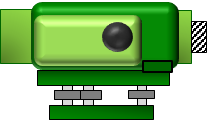## 1. General

Refer to Figure A-1 for illustration of general terms. Some of these were defined in the Basic Principles topic; we will add some elevation terms.Figure A-1 General Elevation Terms

### a. Level line

A line which is perpendicular to the direction of gravity (vertical) along its entire length. Because vertical lines converge, a level line is a curved line.

### b. Level surface

A surface which, like a level line, is everywhere perpendicular to gravity. It is a curved surface.

### c. Vertical datum

A level surface which serves as a datum for vertical distances.

Data can be formal or assumed. Formal data have some defining mathematical parameters and a network of physical marks (see Benchmark) allowing new points to be easily and accurately referenced. Examples of national data include Mean Sea Level (MSL), National Geodetic Vertical Datum of 1929 (NGVD28), and North American Vertical Datum of 1988 (NAVD88). Some regions and cities created and used local formal data before national data were widely available. An assumed datum is created for convenience and is not dependent on a formal datum. Elevations based on an assumed datum are correct relative to each other but not to elevations on other data.

### d. Elevation

The vertical distance from a vertical datum.

### e. Benchmark (BM)

A physical structure whose elevation with respect to a datum is known. It serves as a local reference to a datum allowing us to quickly determine elevations based on that datum.

## 2. Instrument

### a. Level Instrument

A Level is a telescope-based surveying instrument used to establish elevations. It has a gravity-based provision, a bubble, to orient the telescope.Figure A-2 Different Level Instruments

### b. Instrument Set Up

On a perfectly adjusted level, the Line of Sight (LoS) is perpendicular to the instrument's Vertical Axis (VA). When an instrument's bubble is centered, the VA coincides with a vertical line and the LoS is horizontal, Figure A-3.Figure A-3 Properly Oriented Level

At the instrument location, a horizontal line and level line coincide, Figure A-4. The further from the instrument, the greater the lines' separation.Figure A-4 Instrument Setup

## 3. Level Network

A level network (or level circuit) is a series of points whose elevations are to be determined as a single surveying project.

### a. Configuration

Configuration has to do with the physical network design, Figure A-5. A level network is either a:

Link - the network begins and ends on different points, or,

Loop - the network closes back on itself.Figure A-5 Network Configuration

### b. Type

Type has to do with the mathematical network design, Figure A-6. A level network is either:

Open - begins and ends on points whose elevations are either unknown or not known relative to each other on the same datum, or,

Closed - begins and ends on the same point or begins on one BM and ends on another; both BMs are referenced to the same datum.Figure A-6 Network Type
Hits: 17347